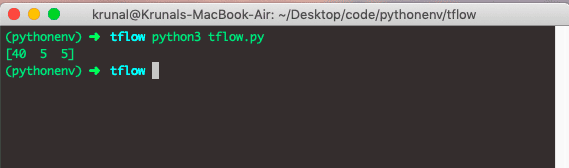# How To Build Simple Model In TensorflowHow To Build Simple Model In Tensorflow - In this tutorial, I will show you How To Build Simple Model In Tensorflow. Tensorflow Framework is the popular framework to design a neural network in Machine Learning.

Tensorflow is created at Google. It is an open source machine learning framework for everyone. TensorFlow is an open source library for high-performance numerical computation. Tensorflow has a flexible architecture that allows easy deployment of calculation across a variety of platforms like CPUs, GPUs, TPUs, and from desktops to clusters of servers to mobile and edge devices. It is initially developed by researchers and engineers from the Google Brain team within Google’s AI organization, and it comes with strong support for machine learning and deep learning, and the flexible numerical computation core is used across many other scientific domains.

## How To Build Simple Model In Tensorflow

If we want to work with Tensorflow then first, we need to define the tensorflow graph. In this model, we will define two tensors or nodes, and then we add those nodes. Now, let’s dive into what is tensors.

## Tensors in Tensorflow

TensorFlow, as the name indicates, is the framework to define and run computations involving tensors. The tensor is the generalization of vectors and matrices to potentially higher dimensions. Internally, TensorFlow represents tensors as n-dimensional arrays of base datatypes.

When writing a TensorFlow program, the primary object you manipulate and pass around is the tf.Tensor. The tf.Tensor *object represents a partially defined computation that will eventually produce a value. TensorFlow programs work by first building a graph of *tf.Tensor objects, detailing how each tensor is computed based on the other available tensors and then by running parts of this graph to achieve the desired results.

TensorFlow programs use the tensor data structure to represent all data only tensors are passed between operations in the computation graph. You can think of the TensorFlow tensor as an n-dimensional array or list.

For example, a scaler is a tensor, a vector is a tensor, and a matrix is a tensor. A tensor has a rank, a shape, and a static type so that a tensor can be represented as the multidimensional array of numbers.

## Tensorflow Example

Okay, now, if you do not know how to install and configure the Tensorflow on virtualenv then check out my post on how to install tensorflow on this blog.

Okay, now we will create a file called *tflow.py *and write the following code inside that file.

``````# app.py

import tensorflow as tf
import os

os.environ['TF_CPP_MIN_LOG_LEVEL'] = '2'

X = tf.placeholder(tf.int32, name='X')
Y = tf.placeholder(tf.int32, name='Y')

with tf.Session() as session:
X: [19, 1, 2],
Y: [21, 4, 3]
})
print(result)
``````

In the above code, first, we have imported the *tensorflow *and *os module *from the Python library.

We have used the *os *module’s *environ attribute *to disable the logs which are unnecessary for this demo model. If you want that log in the output, then you can comment that statement.

Then we have created the computational graph with two nodes using *a placeholder *and defined their datatypes and names.

So, we have two tensors up to now which is X and Y.

Then we are adding those tensors using tensorflow’s add method and get the third node which is an add node.

If we want to execute operation in the Graph, we need to use sessions.

We can create a session in tensorflow using *with tf.Session as session *code.

Next step is that we have called the run method on the session, which takes the two arguments.

2. data

We have used the dictionary data, which has two nodes, X and Y. So we will add those and generate a new node with the session.

Go to the terminal and run the following command.

``````python3 tflow.py
``````

The output of the above code is following.So, we have built a tensorflow model, which can add two nodes and gives the output node.

Here, the computation is to add the nodes and nothing complicated. But in real life application, there are lots of variables and iterables to go through and finally predict the future value.

Finally, How To Build Simple Model In Tensorflow Tutorial With Example is over.

## Machine Learning Tutorial with Python, Jupyter, KSQL and TensorFlow

Machine Learning With Python, Jupyter, KSQL, and TensorFlow. This post focuses on how the Kafka ecosystem can help solve the impedance mismatch between data scientists, data engineers and production engineers.

## Machine Learning, Data Science and Deep Learning with Python

Complete hands-on Machine Learning tutorial with Data Science, Tensorflow, Artificial Intelligence, and Neural Networks. Introducing Tensorflow, Using Tensorflow, Introducing Keras, Using Keras, Convolutional Neural Networks (CNNs), Recurrent Neural Networks (RNNs), Learning Deep Learning, Machine Learning with Neural Networks, Deep Learning Tutorial with Python

## TensorFlow Vs PyTorch: Comparison of the Machine Learning Libraries

Libraries play an important role when developers decide to work in Machine Learning or Deep Learning researches. In this article, we list down 10 comparisons between TensorFlow and PyTorch these two Machine Learning Libraries.